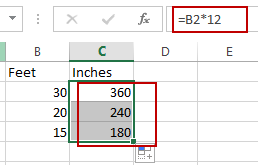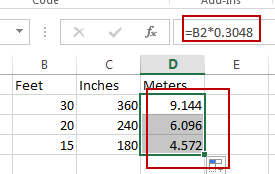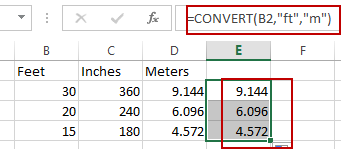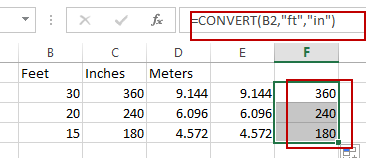# Convert measurements

This post will guide you how to convert feet to inches in Excel. How to convert feet to meters in Excel. How to convert fee to inches or meters with CONVERT function in Excel.

## Convert Feet to Inches with a Formula

If you want to convert feet to inches with a calculator, you need to multiply feet by 12. As one foot is equal to 12 inches. So you can use the following formula to convert the measurement unit from feet to inches:

`=B2 * 12`

Type this formula into cell C2, and press Enter key in your keyboard, and then drag the AutoFill Handle over other cells to apply this formula.If you want to convert feet to meters, you can multiply feet by 0.3048. And one foot is equal to 0.3048 meters. So you can convert feet to meters with the following formula:

`=B2*0.3048`

Type this formula into cell D2, and then press Enter key.## Convert Feet to Meters with Convert Function

You can also use a formula based on the CONVERT function to convert feet to meters in Excel. Just use the following formula:

`=CONVERT(B2,"ft","m")`If you want to convert feet to inches, you can use the following formula:

`=CONVERT(B2,"ft","in")`